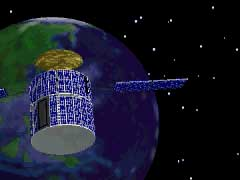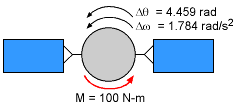Ch 7. Rigid Body Energy Methods Multimedia Engineering Dynamics Rot. Work & Energy Conservation of Energy
 Chapter - Particle - 1. General Motion 2. Force & Accel. 3. Energy 4. Momentum - Rigid Body - 5. General Motion 6. Force & Accel. 7. Energy 8. Momentum 9. 3-D Motion 10. Vibrations Appendix Basic Math Units Basic Equations Sections Search eBooks Dynamics Fluids Math Mechanics Statics Thermodynamics Author(s): Kurt Gramoll ©Kurt GramollDYNAMICS - CASE STUDY SOLUTIONProblem GraphicProblem Diagram The Principle of Work and Energy for a rotating body can be applied to this problem using      T1 + ΣU1-2 = T2 The applied work for a constant moment is      U = M θ = (100 N-m) (4.459 rad)         = 445.9 N-m Now the input energy is known, the change in kinetic energy needs to be determined,      Tstart = T1 = 0      Tfinish = T2 = 1/2 m vG2 + 1/2 IG ω2               = 0 + 0.5 IG (1.784 rad/s)2              = (1.591 /s2) IG Equating the work done to the change in kinetic energy gives      0 + 445.9 N-m = (1.591 /s2) IG Solving for IG gives      IG = Iz = 280.3 kg-m2

Practice Homework and Test problems now available in the 'Eng Dynamics' mobile app
Includes over 400 problems with complete detailed solutions.
Available now at the Google Play Store and Apple App Store.